# D.1 Non-Interacting FERMIons

The HAMILTONian for non-interacting electrons (FERMIons) in momentum representation is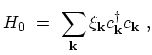(D.1)

where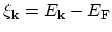is the single-particle energy measured with respect to the FERMI energy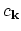and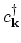are the FERMIon annihilation and creation operators, respectively (Appendix A). The time-evolution of the annihilation operator in the HEISENBERG picture is (Appendix B)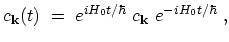(D.2)

so the operator obeys the equation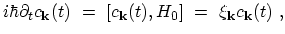(D.3)

which has the solution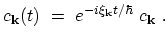(D.4)

The creation operator for FERMIons is the just the HERMITian conjugate of, i.e.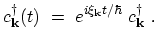(D.5)

The non-interacting real-time GREEN's functions (Section 3.7.1) for FERMIons in momentum representation are now given by(D.6)

where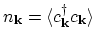is the average occupation number of the state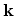. The GREEN's functions depend only on time differences. One usually Fourier transforms the time difference coordinate,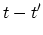, to energy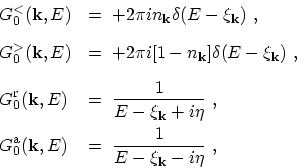(D.7)

where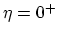is a small positive number. Assuming that the particles are in thermal equilibrium one obtains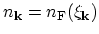, where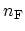is the FERMI-DIRAC distribution function (Appendix C.1). The result (D.7) shows that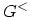and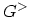provide information about the statistics, such as occupation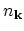or un-occupationof the states, and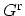and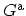provide information about the states regardless of their occupation. The spectral function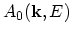for FERMIons is therefore defined as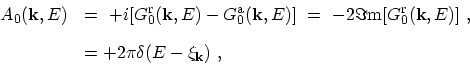(D.8)

where the following relation is used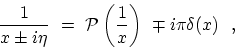(D.9)

where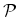indicates the principal value. Under equilibrium the lesser and greater GREEN's functions can be rewritten as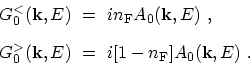(D.10)

M. Pourfath: Numerical Study of Quantum Transport in Carbon Nanotube-Based Transistors